Tamilnadu State Board New Syllabus Samacheer Kalvi 12th Chemistry Guide Pdf Chapter 5 Coordination Chemistry Text Book Back Questions and Answers, Notes.

## Tamilnadu Samacheer Kalvi 12th Chemistry Solutions Chapter 5 Coordination Chemistry

### 12th Chemistry Guide Coordination Chemistry Text Book Questions and Answers

Part – I Text Book Evaluation

I. Choose the correct answer

1. The sum of primary valence and secondary valance of the metal M in the complex [M(en))2 (Ox)] Cl is L
a) 3
b) 6
c) -3
d) 9
d) 9

2. An excess of silver nitrate is added to 100ml of aO.OlM solution of pentaaquachlori- dochromium (III) chloride. The number of moles of AgCl precipitated would be
a) 0.02
b) 0.002
c) 0.01
d) 0.2
b) 0.002

3. A complex has a molecular formula MSO4Cl.6H2O. The aqueous solution of it gives white precipitate with Barium chloride solution and no precipitate is obtained when it is treated with silver nitrate solution. If the secondary valence of the metal is six, which one of the following correctly represents the complex?
a) [M(H2O)4Cl] SO4.2H2O
b) [M(H2O)6] SO4
c) [M(H2O)5Cl]SO4.H2O
d) [M (H2O)3Cl] SO4.3H2O
c) [M(H2O)5Cl]SO4.H2O4. Oxidation state of Iron and the charge on the ligand NO in [Fe (H2O)5 NO] SO4 are
a) +2 and 0 respectively
b) +3 and 0 respectively
c) +3 and -1 respectively
d) +1 and +1 respectively
d) +1 and +1 respectively

5. As per IUPAC guidelines, the name of the complex [Co(en)2 (ONO)Cl] Cl is
a) chlorobisethylenediaminenitritocobalt(III) chloride
b) chi or id obis (e thane-1, 2-diamine) nitrito K-Ocobaltate(III) chloride
c) chloridobis (ethane-1, 2-diammine) nitrito K -Ocobalt(II) chloride
d) chloridobis (ethane-1, 2-diamine) nitrito K -Ocobalt(III) chloride
d) chloridobis (ethane-1,2-diamine) nitro K -Ocobalt(III) chloride

6. IUPAC name of the complex K3[Al(C2O4)3] is
a) potassiumtrioxalatoaluminium(III)
b) potassiumtrioxalatoaluminate(II)
c) potassiumtrisoxalatoaluminate(III)
d) potassiumtrioxalatoaluminate(III)
d) potassiumtrioxalatoaluminate(III)

7. A magnetic moment of 1.73BM will be shown by one among the following (NEET)
a) TiCl4
b) [CoCl6]4-
c) [CU(NH3)4]2+
d) [Ni(CN)4]2-
c) [CU(NH3)4]2+

8. Crystal field stabilization energy for high spin d5 octahedral complex is
a) -0.6∆0
b) 0
c) 2(P – ∆0)
d) 2(P + ∆0)
b) 0

9. In which of the following coordination entities the magnitude of ∆0 will be maximum?
a) [Co(CN)6]3-
b) [Co(C2O4)3]3-
c) [Co(H2O)6]3+
d) [Co(NH3)6]3+
a) [Co(CN)6]3-

10. Which one of the following will give a pair of enantiomorphs?
a) [Cr(NH3)6][Co(CN))6]
b) [Co(en)2Cl2]Cl
c) [Pt(NH3)4][FtCl4]
d) [CO(NH3)4Cl2]NO2
b) [Co(en)2Cl2]Cl

11. Which type of isomerism is exhibited by [Pt(NH3)2Cl2]?
a) Coordination isomerism
c) Optical isomerism
d) Geometrical isomerism
d) Geometrical isomerism

12. How many geometrical isomers are possible for [Pt(Py)(NH3)(Br)(Cl)]?
a) 3
b) 4
c) 0
d) 15
a) 3

13. Which one of the following pairs represents linkage isomers?
a) [Cu(NH3)4] [PtCl4] and [Pt (NH3)4] [CuCl4]
b) [Co(NH3)5 (NO3)] SO4 and [CO(NH3)5 (ONO)]
c) [Co(NH3)4 (NCS)2] Cl and [Co(NH3)4 (SCN)2]Cl
d) both (b) and (c)
Ans:
c) [Co(NH3)4 (NCS)2] Cl and [Co(NH3)4 (SCN)2]Cl14. Which kind of isomerism is possible for a complex [Co(NH3)4Br2]Cl? (PTA -3)
a) geometrical and ionization
b) geometrical and optical
c) optical and ionization
d) geometrical only
a) geometrical and ionization

15. Which one of the following complexes is not expected to exhibit isomerism?
a) [Ni(NH3)4 (H2O)2]2+
b) [Pt(NH3)2Cl2]
c) [Co(NH3)5SO4]Cl
d) [FeCl6 ]3-
d) [FeCl6]3-

16. A complex in which the oxidation number of the metal is zero is
a) K4 [Fe(CN)6]
b) [Fe(CN)3 (NH3)3]
c) [Fe(Co)5]
d) both (b) and (c)
c) Fe(CO)s]

17. Formula of tris(ethane-l,2-diamine) iron (II) phosphate
a) [Fe(CH3 – CH(NH2)2)3](PO4)3
b) [Fe(H2N – CH2 – CH2 – NH2)3] (PO4)
c) [Fe(H2N-CH2-CH2-NH2)3](PO4)2
d) [Fe(H2N-CH2-CH2-NH2)3]3(PO4)2
d) [Fe(H2N-CH2-CH2-NH2)3]3(PO4)2

18. Which of the following is paramagneticin nature? (PTA -5)
a) [Zn(NH3)4]2+
b) [CO (NH3)6]3+
c) [Ni(H2O)6]2+
d) [Ni (CN)4]2-
c) [Ni(H2O)6]2+

19. Fac-mer isomerism is shown by
a) [Co (en)3]3+
b) [Co(NH3)4(Cl)2]+
c) [Co (NH3)3 (Cl)3]
d) [Co (NH3)5 Cl]SO4
c) [Co (NH3)3 (Cl)3]

20. Choose the correct statement.
a) Square planar complexes are more stable than octahedral complexes
b) The spin only magnetic moment of [Cu (Cl)4]42- is 1.732 BM and it has square planar structure.
c) Crystal field splitting energy (∆o) of [FeF6]4- is higher than the (Ao) of [Fe (CN)6]4
d) Crystal field stabilization energy of [V(H2O)f6]2+ is higher than the crystal field stabilization of [Ti(H2O)6]2+
d) Crystal field stabilization energy of [V(H2O)f6]2+ is higher than the crystal field stabilization of [Ti(H2O)6]2+

II. Answer the following questions

Question 1.
Write the IUPAC names for the following complexes. (PTA -3)

1. Na2 [Ni (EDTA)]
2. [Ag(CN)2]
3. [Co(en)3]2 (SO4)3
4. [Co (ONO) (NH3)5]2+
5. [Pt(NH3)2 Cl(NO2)]

1. Sodium 2, 21, 211, 2111-(ethane-1, 2-diyldinitrilo) tetra acetato nickelate (II)
2. Dicyanido k-c argentate (I) ion
3. Tris (ethane -1, 2 – diamine) cobalt (III) sulphate
4. Pentaammine nitrito _ kO cobalt (III) ion
5. Diammine chlorido nitrito – kN Platinum (II)Question 2.
Write the formula for the following coordination compounds.

1. Potassiumhexacyanidoferrate(II)
2. pentacarbonyl iron(O)
3. pentaamminenitrito -k -Ncobalt(III)ion
4. hexaamminecobalt(III) sulphate
5. sodium tetra fluoridodi hydroxidochromate (III)

1. K4[Fe(CN)6]
2. [Fe(CO)5]
3. [CO(NH3)5 (NO2)]2+
4. [CO[NH3)6]2 (SO4)3
5. Na3[CrF4(OH)2]

Question 3.
Arrange the following in order of increasing molar conductivity
i) Mg[Cr(NH3)(Cl)5]
ii) [Cr(NH3)5Cl]3[CoF6]2
iii) [Cr(NH3)3Cl3]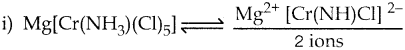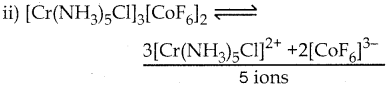iii) [Cr(NH3)3Cl3] – Neutral complex – No ions Molar conductivity increases as the number of ions increase
∴ Molar conductivity is in the increasing order. [Cr(NH3)3Cl3] < Mg (Cr(NH3)Cl5] < [Cr(NH3)5Cl]3[CoF6]2

Question 4.
Give an example of a coordination compound used in medicine and two examples of biologically important coordination compounds.
Medical uses of coordination compounds:

1. Ca-EDTA chelate is used in the treatment of lead and radioactive poisoning. That is for removing lead and radioactive metal ions from the body.
2. Cis-platin is used as an antitumor drug in cancer treatment.

Biological importance of coordination compounds:

1. A red blood corpuscles (RBC) is composed of heme group, which is Fe2+ Porphyrin complex.it plays an important role in carrying oxygen from lungs to tissues and carbon dioxide from tissues to lungs.

2. Chlorophyll, a green pigment present in green plants and algae, is a coordination complex containing Mg2+ as a central metal ion surrounded by a modified Porphyrin ligand called corrin ring. It plays an important role in photosynthesis, by which plants convert CO, and water into carbohydrates and oxygen.

3. Vitamin B12 (cyanocobalamin) is the only vitamin consist of a metal ion. it is a coordination complex in which the central metal ion is CO+ surrounded by Porphyrin ligand.

4. Many enzymes are known to be metal complexes, they regulate biological processes. For example, Carboxypeptidase is a protease enzyme that hydrolytic enzyme important in digestion contains a zinc ion coordinated to the protein.

Question 5.
Based on VB theory explain why [Cr(NH3)6]3+ is paramagnetic, while [Ni(CN)4]2- is diamagnetic.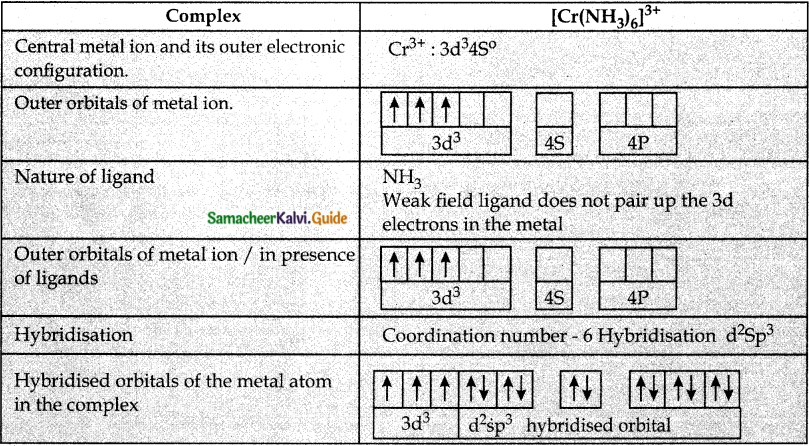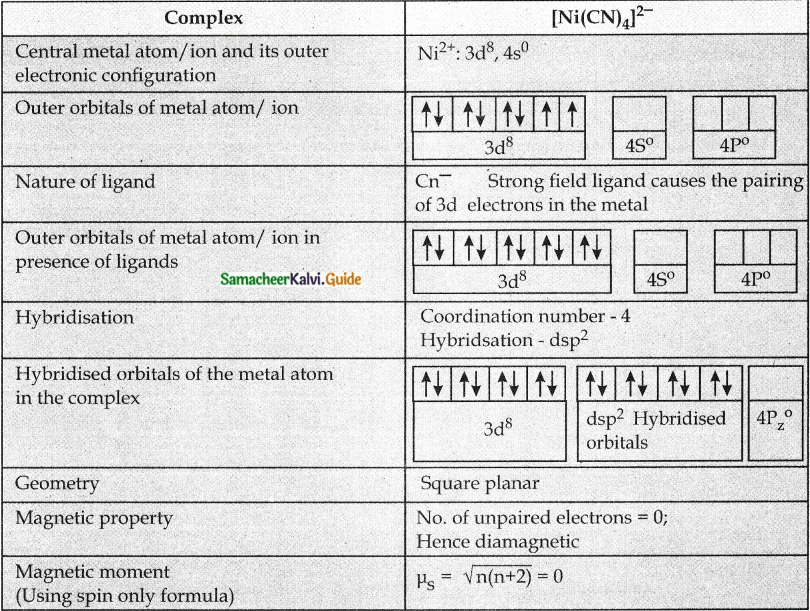Question 6.
Draw all possible geometrical isomers of the complex [Co(en)2Cl2]+ and identify the optically active isomer.Question 7.
[Ti (H2O)6]3+ is coloured, while [Sc (H2O)6]3+ is colourless – Explain. (PAT -4; MARCH 2020)

• In [Ti (H2O)6]3+ the – outer electronic configuration of metal ion Ti3+ is 3d¹.
• This single electron present in lower energy t2g orbitals in the octahedral aqua ligand field absorbs light and excited to higher energy eg level.
• This is known as d-d transition.
• For this excitation absorption maximum is at 20000 cm-1 corresponding to the CFSE ∆0 239.7 KJ mol-1
• The transmitted colour associated with this absorption in purple and hence the complex appears purple in colour. [Ti(H20)6]3+
• But in [Sc(H2O)6]3+, the outer electronic configuration of metal ion Sc3+ is 3d°
• Since there is no electron in d orbital, d-d transition is not possible.
• Hence [Sc(H2O)6]3+ is colourless.

Question 8.
Give an example for complex of the type [Ma2b2C2] where a, b, c are monodentate ligands and give the possible isomers.

• Example for the complex of the type [Ma2b2c2] is [Cr(NH3)2 (H2O)2Br2]+
• There are totally 6 isomers possible Geometrical isomers – 5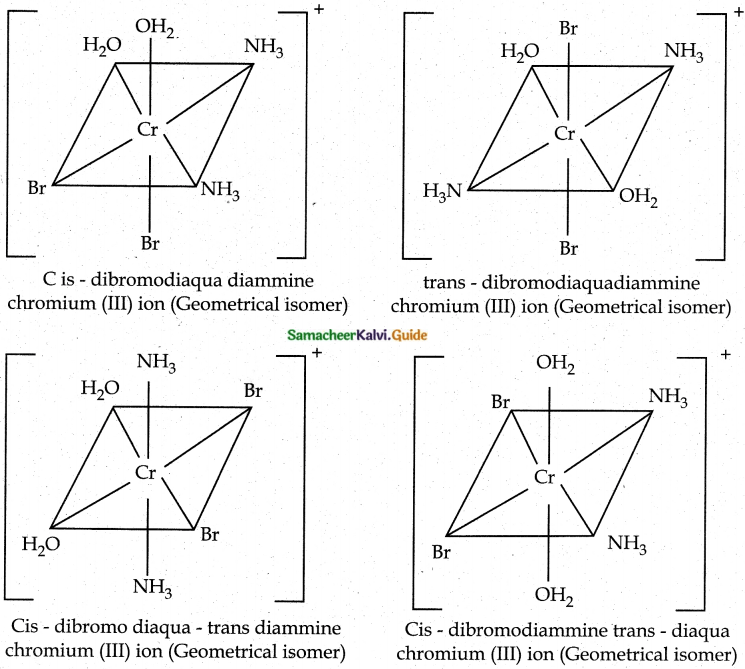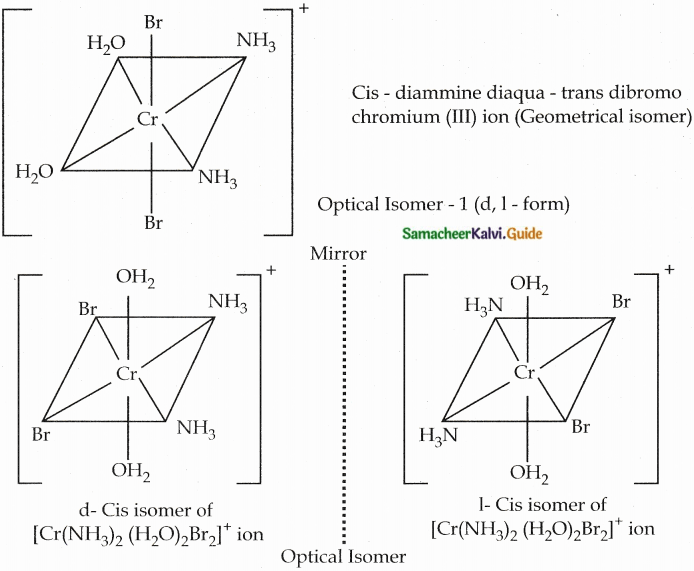Question 9.
Give one test to differentiate [Co(NH3)5Cl]SO4 and [Co(NH3)5 SO4]Cl

• [CO(NH3)5Cl]SO4 → [CO(NH3)5Cl]+2 + SO42-
• [CO(NH3)5SO4] Cl → [CO(NH3)5Cl] SO4

1. Aqueous solution of (a) gives sulphate ion. When the addition of BaCL, solution (a) gives a white precipitate of BaS04. But (b) does not give any precipitate.

2. Aqueous solution of (b) gives chloride ion. When the addition of AgNO3 solution (b) gives curdy white precipitate of AgCl. But (a) does not give any precipitate.

Question 10.
In an octahedral crystal field, draw the figure to show the splitting of d orbitals.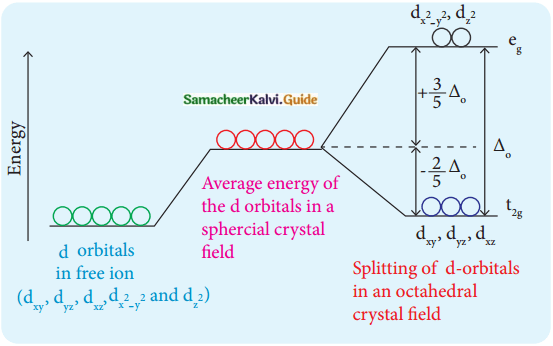Question 11.
What is linkage isomerism? Explain with an example.Linkage isomerism arises when an ambidentate ligand is bonded to the central metal atom/ion through either of its two different donor atoms.
(eg) [Co(NH3)5NO2]2+
[Co(NH3)5NO2]2+
Pentaammine nitrito – kN Cobalt (III) ion – Nattached [CO(NH3)5ONO]2+
Pentaammine nitrito – kO Cobalt (III) ion – O attached

Question 12.
Classify the following ligand based on the number of donor atoms,
a) NH3
b) en
c) ox2-
d) triaminotriethylamine
e) pyridine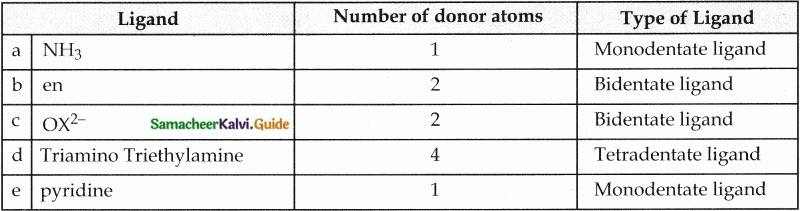Question 13.
Give the difference between double salts and coordination compounds.

 Double Salts Coordination Compounds 1. Lose their identity Do not lose llieii identity 2. Dissociate into their constituent simple ions in solutions Never dissociate to give simple ions. 3. (eg) Mohr’s salt FeSO4(NH4) 2 SO4.6H2O K3[Fe(SCN)6] 4. Answer the tests for simple ions Fe2+, NH4+,SO42- -ions. Does not answer for simple ions Fe3+, SCN–

Question 14.
Write the postulates of Werner’s theory?
In a complex, every metal atom possesses two types of valency.
(i) Primary Valency
(ii) Secondary Valency

 Primary Valency Secondary Valency 1. Refers to the Oxidation State of metal ion Refers to the Coordination number of metal ion 2. Always satisfied by negative ions Satisfied bv negative ions, neutral molecules or positive ions. 3. These ions are generally written outside the bracket are called counter ions. These ions written inside the bracket are called ligands. 4. The outer sphere in which these ions present are called ionisation sphere. The inner sphere in which these ions present are called coordination sphere 5. The groups present in this sphere are loosely bound to the central metal ion and can be separated into ions. The groups present in this sphere are firmly attached to the central metal atom and can not be separated into ions. 6. This Valency is ionisable This valency is non-ionisable. 7. This valency is non-directional This valency is directional and determines the geometry of the complex.

15. Why tetrahedral complexes do not exhibit geometrical isomerism.
Cis-trans isomerism is not possible in tetrahedral complexes because all the four ligands are adjacent to one another [Ma2b2]Question 16.
Explain optical isomerism in coordination compounds with an example.

• Coordination compounds which possess chirality exhibit optical isomerism similar to organic compounds.
• The pair of two optically active isomers which are mirror images of each other are called enantiomers.
• Their solutions rotate the plane of the plane polarised light.
• If the rotation is in the clockwise direction the isomer is called dextro rotatory ‘d’ form.
• If the rotation is in the anti-clockwise direction the isomer is called laevo rotatory T form.
• The octahedral complexes exhibit optical isomerism.
• Examples: [CoCl2(en)2]+

Two Cis isomers are optically active. One trans isomer is optically inactiveQuestion 17.
What are hydrate isomers? Explain with an example. (PTA- 6; March 2020)

• The exchange of free solvent molecules such as water, ammonia, alcohol etc., in the crystal lattice with a ligand in the coordination entity will give Solvate isomers.
• If the solvent molecule is water, then these isomers are called hydrate isomers.
• Example: CrCl3.6H2O has three hydrate isomers
 Complex Colour Number of Chloride ions in solutions [Cr(H2O)6]Cl3 Violet 3 [Cr(H2O)5Cl]Cl2.H2O Pole green 2 [Cr(H2O)4Cl2]Cl.2H2O Dark green 1

Question 18.
What is crystal field splitting energy?
The orbitals lying along the axes dx²-y² and dz² orbitals will experience strong repulsion and raise in energy to a greater extent than the orbitals with lobes directed between the axes (dxy, dyz and dzx). Thus the degenerated orbitals now split into two sets and the process is called crystal field splitting.Question 19.
What is crystal field stabilization energy? (CFSE)? (PTA -1)
Crystal field stabilisation energy (CFSE) is defined as the energy difference of electronic configurations in the ligand field (ELF) and the isotropic field/barycentre (Eiso).
CFSE (∆Eo) = {FLF}-{Eiso} = {[nt2g(-0.4)+neg(0.6)] ∆o + npP} – {n’p P}
Where
nt2g = No. f electrons in t2g orbitals
neg = No. of electrons in eg orbitals
np = No. of electron in the ligand field
p = No. of electron pairs in the isotropic field (barycentre)
P = pairing energy

Question 20.
A solution of [Ni(H2O)6]2+ is green, whereas a solution of [Ni(CN)4]2- is colorless – Explain.

• In [Ni(H2O)6]2+, the Ni2+ ion has two unpaired electrons that do not pair up in presence of weak ligand H2O.
• Hence d-d-the transition is possible.
• Red colour is absorbed and complementary colour green is emitted. So it is green in colour.
• In [Ni(CN)4]2-, the Ni2+ ion has no unpaired electrons because of the strong ligand CN pairs up the electrons.
• Hence no d-d transition is possible.
• So [Ni(CN)4]2- is colourless

Question 21.
Discuss briefly the nature of bonding in metal carbonyls. (PTA – 2)

• In metal carbonyls, the bond between metal atom and the carbonyl ligand consists of two components.
• The first component is an electron pair donation from the carbon atom of carbonyl ligand into a vacant d-orbital of central metal atom.
• This electron pair donation forms M←CO sigma bond.
• This sigma bond formation increases the electron density in metal d-orbitals and makes the metal electron-rich.
• In order to compensate for this increased electron density, a filled metal d-orbital interacts with the empty π* orbital on the carbonyl ligand and transfers the added electron density back to the ligand.
• This second component is called n-back bonding.
• Thus in metal carbonyls, electron density moves from ligand to metal through sigma bonding and from metal to ligand through pi bonding.
• This synergic effect accounts for a strong M←CO bond in metal carbonyls.
• This is shown diagrammatically as follows.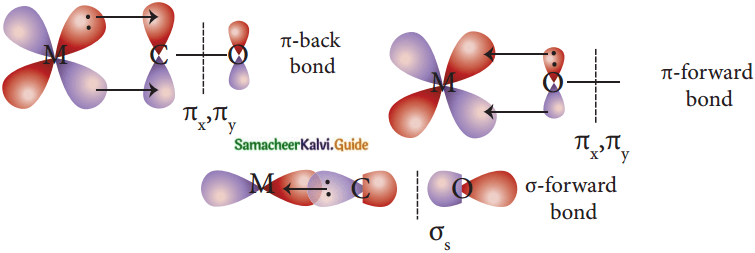Question 22.
What is the coordination entity formed when an excess of liquid ammonia is added to an aqueous solution of copper sulphate?
When excess liquid ammonia is added to an aqueous solution of copper sulphate tetraammine copper (II) sulphate is formed.
CuSO4 + 4NH3 → [Cu(NH3)4]SO4. The Coordination entity is [Cu(NH3)4]2+.

Question 23.
On the basis of VB theory explain the nature of bonding in [Co(C2O4)3]3-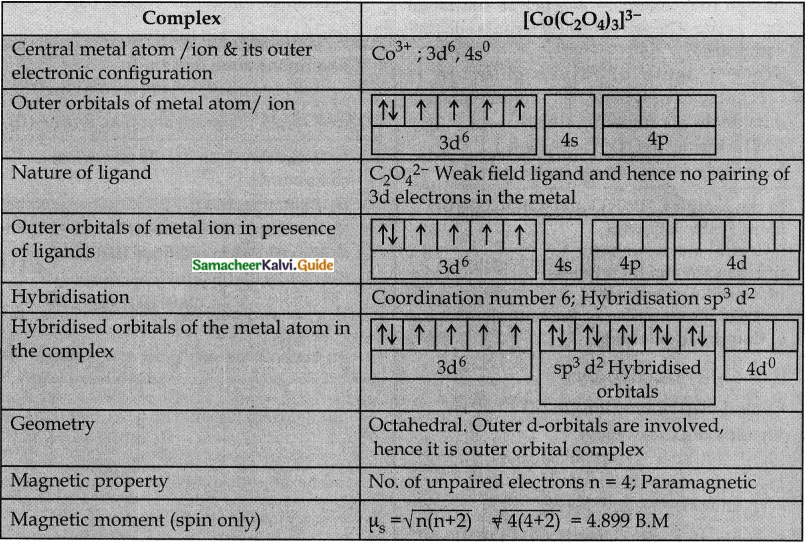Question 24.
What are the limitations of VB theory?
Limitations of VB – Theory:
1. It does not explain the colour of the complex

2. It considers only the spin only magnetic moments and does not consider the other components of magnetic moments.

3. It does not provide a quantitative explanation as to why certain complexes are inner orbital complexes and the others are outer orbital complexes for the same metal. For example, [Fe(CN)6]4- is diamagnetic (low spin) whereas [Fe(CN)6]4- is paramagnetic (high spin).

Question 25.
Write the oxidation state, coordination number, nature of ligand, magnetic property and electronic configuration in octahedral crystal field for the complex K4[Mn(CN)6].

 (i) Oxidation State +2 (Mn2+) (ii) Coordination Number 6 (iii) Nature of ligand Strong field (N–) (iv) Magnetic property Paramagnetic (v) Electronic configuration t52g, e0g

III. Evaluate yourself

Question 1.
When a coordination compound CrCl3.4H2O is mixed with silver nitrate solution, one mole of silver chloride is precipitated per mole of the compound. There are no free solvent molecules in that compound. Assign the secondary valence to the metal and write the structural formula of the compound.
Since 1 mole of silver chloride is precipitated only one Cl ion is present outside the coordination sphere.
∴ The formula is [Cr(H2O)4Cl2]Cl
Secondary valence of the metal is 6

Question 2.
In the complex, [Pt(NO2)(H2O)(NH3)2]Br, identify the following
i. Central metal atom/ion
ii. Ligand(s) and their types
iii. Coordination entity
iv. Oxidation number of the central metal ion
v. Coordination number
[Pt(NO2)(H2O)(NH3)2]Br
Central metal atom/ ion : Pt (II)
Ligands and their types :
NO2 nitrito-k N – Anionic ligand
H2O aqua – Neutral ligand
NH3 ammine – Neutral ligand
Coordination entity: [Pt(NO2)(H2O)(NH3)2]+
Oxidation number of the central metal ion x + (-1) + 0 + 2 (0) = +1; x = +2
Coordination number = 4

Question 3.
Write the IUPAC name for the following compounds.
(i) K2[Fe(CN)3(Cl)2(NH3)]
(ii) [Cr(CN)2(H2O)4][Co(OX)2(en)]
(iii) [Cu(NH3)2Cl2]
(iv) [Cr(NH3)3(NC)2(H2O)]+
(v) [Fe(CN)6]4-
(i) K2[Fe(CN)3(Cl)2(NH3)]
Potassium amminedichloridotricyanido- kC ferrate (III)
(ii) [Cr(CN)2(H2O)4][Co(OX)2(en)]
Tetra aqua dicyanido kc chromium (III) (ethane -1,2-diamine) dioxalato cobaltate (III)
(iii) [Cu(NH3)2Cl2]
Diammine dichlorido copper (II)
(iv) [Cr(NH3)3(NC)2(H2O)]+
Triammine aqua dicyanido -kN chromium (III) ion
(v) [Fe(CN)6]4- Hexacyanido-kc ferrate (II) ionQuestion 4.
Give the structure for the following compounds.
(i) diamminesilver(I) dicyanidoargentate(I)
(ii) Pentaammine nitrito-KNcobalt (III) ion
(iii) hexafluorido cobaltate (III) ion
(iv) dichloridobis (ethylenediamine)
Cobalt (III) sulphate
(v) Tetracarbonylnickel (0)
(i) diamminesilver(I) dicyanidoargentate(I) : [Ag(NH3)2] [Ag(CN)2]
(ii) Pentaammine nitrito-KNcobalt (III) ion : (CO(NH3)5 (NO)2]2+
(iii) hexafluorido cobaltate (III) ion : [CoF6]3-
(iv) dichloridobis (ethylenediamine) Cobalt (III) sulphate : [Co(en)2Cl2]2SO4
(v) Tetracarbonylnickel (0): [Ni(CO)4]

Question 5.
A solution of [Co(NH3)4I2]Cl, when treated with AgNO3, gives a white precipitate. What should be the formula of isomer of the dissolved complex that gives yellow precipitate with AgNO3. What are the above isomers called?

• Since yellow precipitate is obtained with AgN03 iodide ion is present outside the coordination sphere.
• Hence the formula is [Co(NH3)4I Cl]I
• This complex is an ionisation isomer of the complex [Co(NH3)4I2]Cl

Question 6.
Three compounds A, B and C have empirical formula CrCl3.6H2O. They are kept in a container with a dehydrating agent and they lost water and attaining constant weight as shown below.Question 7.
Indicate the possible type of isomerism for the following complexes and draw their isomers,
i) [Co(en)3][Cr(CN)6]
ii) [Co(NH3)5(NO2)]2+
iii) [Pt(NH3)3(NO2)]Cl
i) [Co(en)3][Cr(CN)6] and [Cr(en)3][Co(CN)6] are coordination isomers
ii) [CO(NH3)5(NO2)]2+ and [Co(NH3)5ONO]2+ are linkage isomers
iii) [Pt(NH3)3(NO2)]Cl and [Pt(NH3)3Cl] N02 are ionisation isomers
iv) [Pt(NH3)3(NO2)] and [Pt(NH3)3ONO)]Cl are linkage isomers.

Evaluate yourself: 8
Question 1.
Draw all possible stereoisomers of a complex Ca[Co(NH3)Cl(Ox)2]Evaluate yourself: 9
Question 1.
The spin-only magnetic moment of the Tetrachloridomanganate(II)ion is 5.9 BM. On the basis of VBT, predict the type of hybridisation and geometry of the compound.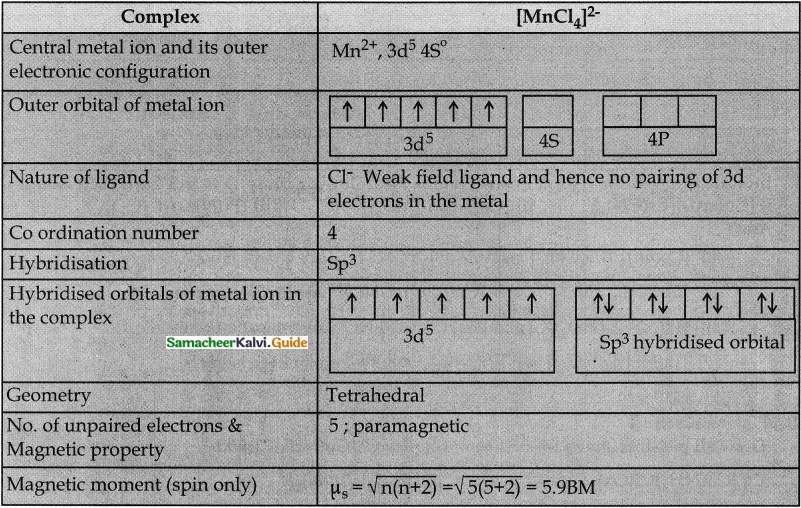Question 2.
Predict the number of unpaired electrons in [C0Cl4]2- ion on the basis of VBT. [C0Cl4]2-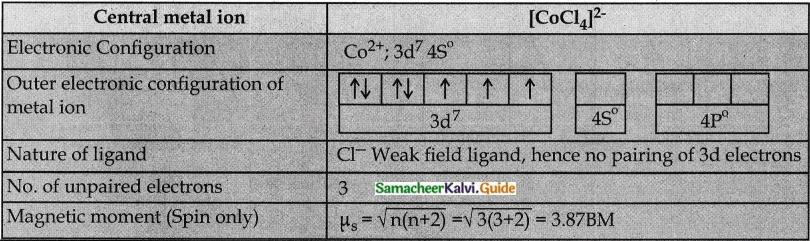Question 3.
A metal complex having composition Co(en)2Cl2Br has been isolated in two forms A and B. (B) reacted with silver nitrate to give a white precipitate readily soluble in ammonium hydroxide. Whereas A gives a pale yellow precipitate. Write the formula of A and B. state the hybridization of Co in each and calculate their spin only magnetic moment. [Co(en)2Cl2Br.
Since B gives white precipitate with AgNO3, it contains free Cl ion outside the Coordination sphere. Hence B is [Co(en)2Cl Br]Cl

Since A gives pale yellow precipitate with AgNO3 it contains free Br ion outside the coordination sphere. Hence A is [Co(en)2Cl2]BrEvaluate yourself: 10
Question 1.
The mean pairing energy and octahedral field splitting energy of [Mn(CN)6]3- are 28,800 cm-1 and 38500 cm-1 respectively. Whether this complex is stable in low spin or high spin?
High spin Mn3+ complex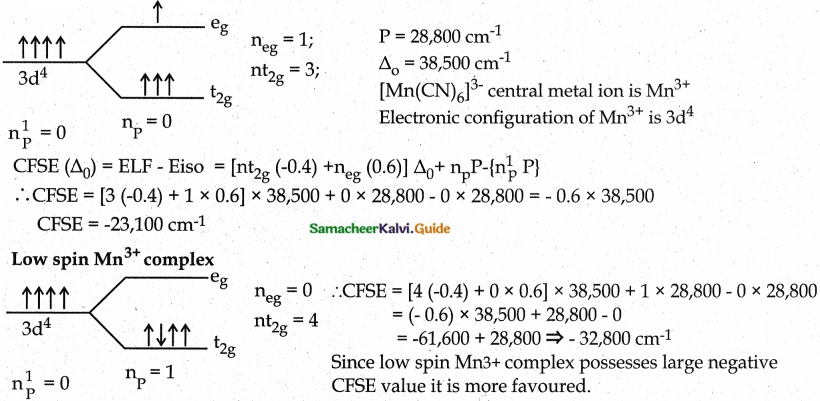Question 2.
Draw energy level diagram and indicate the number of electrons in each level for the complex [Cu(H2O)6]2+. Whether the complex is paramagnetic or diamagnetic?
[Cu(H2O)6]2+Since one unpaired electron is present, the complex is paramagnetic and C.N is 6 the geometry is Octahedral.

Question 3.
For the [CoF6]3- ion the mean pairing energy is found to be 21000 cm-1. The magnitude of ∆0 is 13000cm-1. Calculate the crystal field stabilization energy for this complex ion corresponding to low spin and high spin states. [CoF6]3-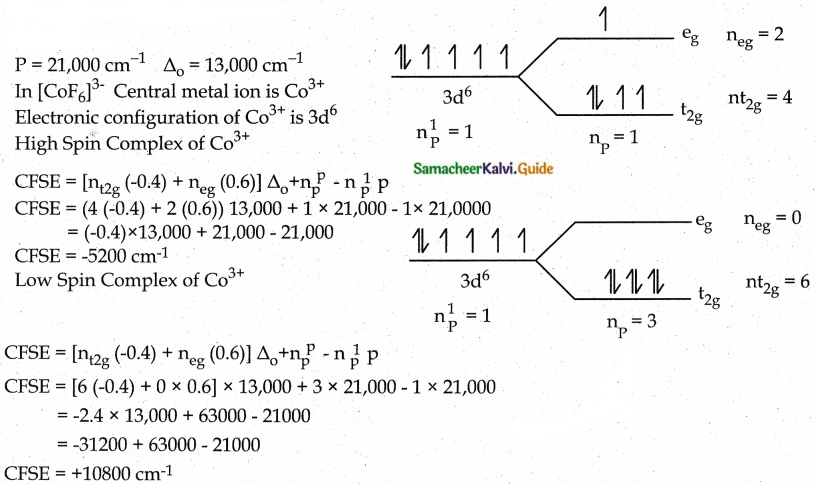Since the high spin complex possesses a large nature CFSE value. It is more favoured.

### 12th Chemistry Guide Coordination Chemistry Additional Questions and Answers

Part II – Additional Questions

I. Choose the best answer.

1. A theory of co-ordination compounds was proposed by …………….
a) Rutherford
b) J.J.Thomson
c) Alfred Werner
d) Neils Bohr
c) Alfred Werner

2. The primary valence of a metal ion refers to …………….
a) atomic number
b) oxidation state
c) atomic mass
d) Co-ordination number
b) oxidation state

3. The secondary valence of a metal ion refers to …………….
a) atomic number
b) oxidation state
c) atomic mass
d) Co-ordination number
d) Co-ordination number

4. Primary valence of a metal ion is always satisfied by …………….
a) Positive ions
b) negative ions
c) neutral molecules
d) all of the above
b) negative ions

5. Among the following complexes, which one shows Zero Crystal field stability: energy (CFSE) is (PTA- 6)
a) [Mn(H2O)3]3+
b) [Fe(H2O)6]33+
c) [Co(H2O)6]2+
d) [CO(H2O)6]3+
b) [Fe(H2O)6]33+

6. The sphere in which the metal ion and ligands are firmly attached is called
a) outer sphere
b) ionization sphere
c) Coordination sphere
d) none of the above
c) Coordination sphere

7. The sphere in which the metal ion and other ions are loosely bound is called
a) Inner sphere
b) Ionization sphere
c) Coordination sphere
d) none of the above
b) ionization sphere

8. The groups which satisfy the secondary valence are called
a) Central metal ion
b) ligands
c) Complexion
d) Complex
b) ligands

9. In a complex a central metal ion acts as a
a) Lewis acid
b) Lewis base
c) Bronsted acid
d) Bronsted base
a) Lewis acid

10. In a complex a ligand acts as a
a) Lewis acid
b) Lewis base
c) Bronsted acid
d) Bronsted base
b) Lewis base

11. In a complex primary valence of a metal ion is
a) ionizable
b) non-ionisable
c) directional
d) Coordination number
a) ionizable

12. In a complex secondary valence of a metal ion is
a) ionizable
b) non-ionisable
c) non-directional
d) Oxidation state
b) non – ionisable

13. In a complex primary valence of a metal ion is
a) non-ionizable
b) directional
c) non-directional
d) Co-ordination number
c) non-directional

14. According to spectrochemical series which of the following ligand produces strongest field and cause maximum splitting? (PTA -1)
a) F
b) CO
c) H2O
d) C
b) CO

15. In the complex K4[Fe(CN)6] the central metal ion is
a) K+
b) Fe2+
c) Fe3+
d) CN
b) Fe2+

16. In the complex K4[Fe(CN)6] the ligand is
a) K+
b) Fe2+
c) Fe3+
d) CN
d) CN17. In the complex K4[Fe(CN)6] the primary valence of the central metal ion is
a) +2
b) +3
c) +4
d) +6
a) +2

18. In the complex K4[Fe(CN)6] the secondary valence of the central metal ion is
a) 2
b) 3
c) 4
d) 6
d) 6

19. The geometry of the complex K4[Fe(CN)6] is
a) Square planar
b) tetrahedral
c) Octahedral
d) trigonal bipyramidal
c) Octahedral

20. K [PtCl3(C2H4)] was called as
a) Wilkinson Catalyst
b) Zeiglar Natta catalyst
c) Magnus’s green salt
d) Zeise’s salt
d) Zeise’s salt

21. Which among the following is not a neutral ligand?
a) aqua
b) ammine
c) Oxalato
d) Pyridine
c) Oxalato

22. Which is a monodentate ligand?
a) Carbonato
b) Oxalato
c) Cyanido
d) en
Ans :
c) Cyanido

23. [Co (NH3)5 NO2]2+ ion exhibits
a) Ionisation isomerism
c) Coordination isomerism
d) solvate isomerism

24. [Pt (NH3)4] [Pd(Cl)4] complex exhibits
a) Ionisation isomerism
c) Coordination isomerism
d) solvate isomerism
c) Coordination isomerism

25. [Cr(NH3)4ClBr] NO2 and [Cr(NH3)4ClNO2] Br are
b) Ionisation isomers
c) Coordination isomers
d) solvate isomers
b) Ionisation isomers

26. Which among the following gives a curdy white precipitate with silver nitrate solution?
a) [Co (NH3)4 Cl Br] Br
b) [Co (NH3)4 Br2] Cl
c) [Cr (NH3)4 Cl Br] NO2
d) [Cr (NH3)4 ClNO2] Br
b) [Co (NH3)4 Br2] Cl27. Which among the following gives three moles of silver chloride with silver nitrate solution?
a) [Cr(H2O)4 Cl2]Cl. 2H2O
b) [Cr(H2O)5 Cl]Cl2. 2H2O
c) [Cr(H2O)6]Cl3
d) [Cr(H2O)3 Cl3]. 3H2O
c) [Cr(H2O)6]Cl3

28. Which among the following gives a white precipitate with barium chloride solution?
a) [Co (NH3)5 Cl] Cl2
b) [Cu(NH3)4] SO4
c) [Ag (NH3)2] Br
d) [Co (NO2)3 (NH3)3]
b) [Cu (NH3)4] SO4

29. According to VBT, in a Coordination complex the bonding between central metal ion and ligands is
a) ionic
b) covalent
c) metallic
d) vander waals force
b) covalent

30. In which of the following complexes the central metal ion undergoes SP3 hybridisation
a) [Ni(CN)4]2-
b) [Pt(NH3)4]2+
c) [NiCl4]2-
d) [Cu (NH3)4]2+
Ans :
c) [[NiCl4]2-

31. If the (n-1) d orbitals are involved in hybridisation, the complex is called as
a) Outer orbital complex
b) Spin paired complex
c) High spin complex
d) Spin free complex
b) Spin paired complex

32. If the n d orbitals are involved in hybridisation, the complex is called as
a) Inner orbital complex
b) low spin complex
c) high spin complex
d) spin paired complex
c) high spin complex

33. Which statement is incorrect?
a) [Ni(CO)4] – Tetrahedral, Paramagnetic
b) [Ni(CN)4]2- – Square planar, diamagnetic
c) [Ni(CO)4] – Tetrahedral, diamagnetic
d) [Ni(Cl)4]2- – Tetrahedral, Paramagnetic
a) [Ni(CO)4] – Tetrahedral, Paramagnetic

34. In [Ni(CO)4], the hybridisation of central metal ion is
a) dsp²
b) sp³
c) d²sp³
d) dsp³
b) Sp³

35. Co-ordination number of Ni in [Ni(C2O4)3]4- is (PTA – 4)
a) 3
b) 6
c) 4
d) 2
b) 6

36. According to CFT, the bonding between central metal ion and ligands is
a) ionic
b) covalent
c) metallic
d) Vanderwaal’s force
a) ionic

37. Which is a strong field ligand?
a) I
b) CN
c) Cl
d) S2-
b) CN

38. Which is a weak field ligand?
a) NO2
b) NH³
c) CO
d) SCN
d) SCN

39. The observed colour of a coordination compound can be explained using
a) Valence bond theory
b) Werner’s theory
c) Crystal field theory
d) Molecular orbital theory
c) Crystal Field theory

40. Which among the following is a mononuclear carbonyl?
a) [C02(C0)8]
b) [Fe3(CO)12]
c) [Fe(CO)5]
d) [Fe2(CO)9]
c) [Fe (CO)5]41. If the instability constant value of [Cu(NH3)4]2+ is 1.0 × 10-12, its stability constant value is:
a) 1.0 × 10-12
b) 1.0 × 1012
c) 12
d) -12
b) 1.0 × 1012

42.

 Complexion Instability constant (∝) [Fe (SCN)]2+ 1.0 × 10-3 [Cu(NH3)4]2+ 1.0 × 10-12 [Ag (CN)2]– 1.8 × 10-19 [Co (NH3)6]3+ 6.2 × 10-36

From the above table which of the following complex is most stable?
a) [Cu (NH3)4]2+
b) [Fe (SCN)]2+
c) [CO(NH3)6]3+
d) [Ag(CN)2]
c) [CO(NH3)6]3+

43. Phthalo blue – a bright blue pigment is a complex of
a) Ni2+
b) Co3+
c) Cu2+
d) Ag+
Ans:
c) Cu2+

44. For removing lead poisoning, the chelating ligand used is
a) DMG
b) EDTA
c) en
d)CO
b) EDTA

45. The process in which coordination complexes are used in the extraction of silver and gold from their ores is
a) Mond’s process
b) Alumino thermic process
c) Mac – Arthur – Forrest cyanide process
d) Bessimerisation
c) Mac- Arthur – Forrest cyanide process

46. Ni ions present in nickel chloride solution is estimated accurately using the ligand
a) DMG
b) EDTA
c) en
d) CO
Ans:
a) DMG

47. Catalyst used for hydrogenation of alkenes is
a) Ziegler – Natta Catalyst
b) Zeise’s Salt
c) Wilkinson’s catalyst
d) Magnus’s green Salt
c) Wilkinson’s Catalyst

48. The catalyst used in the polymerization of ethene is
a) Ziegler – Natta Catalyst
b) Zeise’s Salt
c) Wilkinson’s catalyst
d) Magnus’s green Salt
a. Ziegler – Natta Catalyst49. The complex used as an antitumor drug in cancer treatment is
a) Ca – EDTA Chelate
b) Cisplatin
c) Trans – Platin
d) Cyano cobalamine
b) Cis – Platin

50. RBC is composed of
a) Mg2+
b) Fe2+
c) Fe3+
d) Co+
b) Fe2+

51. In RBC and chlorophyll, the ligand is
a) EDTA
b) DMG
c) Porphyrin
d) en
c) Porphyrin

II. Match the following
Question 1.

 Complex Type i) K2[Ni(CN)4l a) Neutral complex ii) [Co(NH3)5NO2]Cl2_ b) Metal carbonyl iii) [Co(NH3) 3C13] c) anionic complex iv) [Ni(CO4] d) Cationic complex

 Complex Type i) K2[Ni(CN)4l c) anionic complex ii) [Co(NH3)5NO2]Cl2_ d) Cationic complex iii) [Co(NH3) 3C13] a) Neutral complex iv) [Ni(CO4] b) Metal carbonyl

Question 2.

 Isomer Reason i) Coordination isomers a) Exchange of free solvent molecules with ligand ii) Linkage isomers b) Different ions in solution iii) Ionisation isomers c) Interchange of one or more ligands iv) Solvate isomers d) Bonding through different donor atoms

 Isomer Reason i) Coordination isomers c) Interchange of one or more ligands ii) Linkage isomers d) Bonding through different donor atoms iii) Ionisation isomers b) Different ions in solution iv) Solvate isomers a) Exchange of free solvent molecules with ligand

Question 3.

 Carbonyl Type i) [Ni(CO)4] a) Polynuclear carbonyl ii) [Fe3(CO)12] b) Bridged carbonyl iii) Fe2(CO)9 c) Mononuclear carbonyl

 Carbonyl Type i) [Ni(CO)4] c) Mononuclear carbonyl ii) [Fe3(CO)12] a) Polynuclear carbonyl iii) Fe2(CO)9 b) Bridged carbonyl

Question 4.

 Carbonyl Geometry i) Chromium hexacarbonyl a) Tetrahedral ii) Iron pentacarbonyl b) Octahedral iii) Nickel tetra carbonyl c) Trigonal bipyramidal

 Carbonyl Geometry i) Chromium hexacarbonyl b) Octahedral ii) Iron pentacarbonyl c) Trigonal bipyramidal iii) Nickel tetra carbonyl a) Tetrahedral

Question 5.

 Hybridisation Geometry i) sp a) Square planar ii) sp2 b) Octahedral iii) sp3 c) Trigonal bipyramidal iv) dsp2 d) Trigonal planar v) dsp3 e) Lineal vi) d2sp3 f) Tetrahedral

 Hybridisation Geometry i) sp e) Lineal ii) sp2 d) Trigonal planar iii) sp3 f) Tetrahedral iv) dsp2 a) Square planar v) dsp3 c) Trigonal bipyramidal vi) d2sp3 b) Octahedral

III. Pick the odd man out

1. Pick the odd man out w.r.t complexion
a) [CO(NH3)6] Cl3
b) [Fe (H2O)6] Cl2
c) [Cu(NH3)4]SO4
d) K4 [Fe(CN)6]
K4 [Fe(CN)6] – Anionic complex others are cationic complexes

2. Pick the odd man out w.r.t geometry
a) [CO(NH3)6] Cl3
b) [Fe (H2O)6] Cl2
c) [Cu(NH3)4]SO4
d) K4 [Fe(CN)6]
c) [Cu(NH3)4]SO43. Pick the add man out w.r.t primary valence of the metal ion
a) [CO(NH3)6]Cl3
b) K3[Fe(CN)6]
c) [Fe(CO)5]
d) [CO(NH3)3 Cl3]
c) [Fe(CO)5] – Primary valence is zero, in other complexes the primary valence is +3

4. Pick the odd man out w.r.t the nature of the ligand
a) aqua
b) carbonyl
c) nitrosyl
d) nitrato
d) nitrato – negative ligand others are neutral ligand

IV. Pick the wrong statement

Question 1.
i) The secondary valence of a metal ion is satisfied by ligands.
ii) The secondary valence determines the geometry of the complex.
iii) The secondary valence is oxidation state of the metal ion.
iv) If the secondary valence is six, the geometry is tetrahedral.
a) (i) & (ii)
b) (i) & (iii)
c) (ii) & (iii)
d) (iii) & (iv)
d) (iii) & (iv)
Correct statement : (iii) The secondary valence is the coordination number of the metal ion (iv) If the secondary valence is six, the geometry is octahedral.

Question 2.
i) Central metal ion and ligands are bonded through ionic bond.
ii) Central metal ion accepts electron pairs donated by ligands.
iii) Ligands act as lewis acids
iv) Charge on the coordination sphere is the net change inside the coordination sphere.
a) (i) & (ii)
b) (i) & (iii)
c) (ii) & (iii)
d) (iii) & (iv)
b) (i) & (iii)
Correct statement: (i) Central metal ions and ligands are bonded through a coordinate covalent bond, (iii) Ligands act as Lewis bases.

Question 3.
i) Trans [Co Cl2 (en)2]+ is optically active.
ii) In octahedral complexes if nd orbitals are involved in hybridisation, they are called as low spin complexes.
iii) In a complex if all the electrons are paired, it is diamagnetic
iv) Ligands which cause the pairing of electrons are called strong field ligands.
a) (i) &(ii)
b) (i) & (iii)
c) (ii) & (iii)
d) (iii) & (iv)
a) (i) & (ii)
Correct statement:
i) Trans [Co Cl2 (en)2]+ is optically inactive.
ii) In octahedral complexes if nd orbitals are involved in hybridisation, they are called high spin complexes.Question 4.
i) The ligands present on the right side of the spectrochemical series are called strong field ligands.
ii) The ligands present on the left side of the spectrochemical series are called weak field ligands.
iii) CFSE (∆E0 = Eiso – ELF
iv) $$\mu_{S}=\sqrt{n(n-2)}$$
a) (i) & (ii)
b) (i) & (iii)
c) (ii) & (iii)
d) (iii) & (iv)
c) (iii) & (iv)
Correct statement: (iii) CFSE (∆E0) = ELF – Eiso
iv) $$\mu_{s}=\sqrt{n(n+2)}$$

V. Pick the Correct statement

Question 1.
i) The primary valence of a metal ion is positive in most cases and zero in certain cases.
ii) The primary valences are directional
iii) The primary valence is satisfied by ligands in certain cases.
iv) The primary valence is co-ordination number.
a) (i) &(ii)
b) (i) & (iii)
c) (ii) & (iii)
d) (iii) & (iv)
b) (i) & (iii)
Correct statement: (ii) The primary valences are non – directional
(iv) The primary valence is oxidation state.

Question 2.
i) Coordination entity consists of central metal ion and ligands.
ii) In K4[Fe(CN)6], the coordination entity is [Fe(CN)6]4-
iii) The groups present inside the coordination entity can be ionised
iv) The coordination entity is not responsible for the geometry of the complex.
a) (i) & (ii)
b) (i) & (iii)
c) (ii) & (iii)
d) (iii) & (iv)
a) (i) & (ii)
Correct statement : (iii) The groups present inside the coordination entity can not be ionised.
(iv) The coordination entity is responsible for the geometry of the complex.

Question 3.
i) In thiocyanate ligand if sulphur forms a coordination bond with metal, the ligand is named thiocyanato-KN
ii) The ligand NH3 is named amine.
iii) Ethylenediamine is a neutral ligand.
iv) The name of cationic ligand ends with – ium
a) (i) &(ii)
b) (i) & (iii)
c) (iii) & (iv)
d) (i) & (iii)
c) (iii) & (iv)
Correct statement : (i) In thiocyanate ligand if sulphur forms a coordination bond with metal, the ligand is named thiocyanato-kS.
(ii) The ligand NH3 is named as ammine.

Question 4.
i) Linkage isomer is not possible with nitrite ligand.
ii) Coordination isomerism is found in coordination compounds in which both cation and anion are complexions.
iii) Ionisation isomer arises when an ionisable counter ion itself can act as a ligand.
iv) In solvate isomer if the solvent molecule is alcohol it is called a hydrate isomer.
a) (i) &(ii)
b) (i) & (iii)
c) (iii) & (iv)
d) (i) & (iii)
b) (ii) & (iii)
Correct statement : (i)Lingake isomer is possible with nitrite ligand (ii) In solvate isomer if the solvent molecule is water it is called as hydrate isomer.

VI. Assertion and Reason

i) Both A and R are correct, R explains A.
ii) A is correct, R is wrong
iii) A is wrong, R is correct
iv) Both A and R are correct, but R does not explain A.Question 1.
Assertion (A) : [Co(NH3)4 Br2] Cl and [CO(NH3)4 Cl Br] Br are ionisation isomers.
Reason (R) : In solution, they give free Cl and Br ions respectively
i) Both A and R are correct, R explains A.

Question 2.
Assertion (A): Geometrical isomerism exists in homoleptic complexes.
Reason (R): Geometrical isomerism is due to different possible three-dimensional spatial arrangements of the ligands around the central metal atom.
iii) A is wrong, R is correct.
Correct Assertion (A): Geometrical isomerism exists in heteroleptic complexes.

Question 3.
Assertion (A) : According to VBT the ligand → metal bond in a coordination complex, is covalent in nature.
Reason (R) : Mutually shared electrons are provided equally by the central metal atom and the ligand
ii) A is correct, R is wrong Correct Reason: Mutually shared electrons are provided by ligands to the central metal atom.

VII. Two Mark Questions

Question 1.
Write any two medicinal uses of coordination compounds? (PTA – 6)
Ca-EDTA chelate is used in the treatment of lead and radioactive poisoning. That is for removing lead and radioactive metal ions from the body.
cisplatin is used as an antitumor drug in cancer treatment.

Question 2.
What is a central metal ion?
The metal ion which accepts electron pair donated by ligands is called the central metal ion of a complex.
Central metal ions are electron-pair acceptors.
Central metal ions are Lewis acids.Question 3.
What are ligands?
In a complex, the negative ions, neutral molecules positive ions which donate pair of electrons to the central metal ion are called ligands.
Ligands are electron-pair donors.
Ligands are Lewis bases.

Question 4.
[Fe(CN6)]4- and [Fe(H2O)6]2+ are of different colours in dilute solutions, why? (PTA – 2)

• [Fe(CN6)]4- and [Fe(H2O)6]2+ show different colours in dilute solution because
• CN is a strong field ligand and H2O is a weak ligand hence magnitude of CFSE is different
• both CN and H2O absorb same wavelength of energy.
• complexes of weak field ligands are generally colourless
• the sizes of CN and H2O are different hence their colours are also different.

Question 5.
Define coordination number.
The number of ligand donor atoms bonded to a central metal ion in a complex is called the coordination number of the metal.

Question 6.
What is the coordination sphere?
The complexion containing the central metal atom/ion and the ligands attached to it is collectively called a coordination sphere or inner sphere.

Question 7.
What are inert and labile complexes? (PTA – 4)
In some cases, complexes can undergo rapid ligand substitution, such complexes are called labile complexes. However, some complexes undergo ligand substitution very slowly (or sometimes no substitution), such complexes are called inert complexes.

Question 8.
What is d-d transition?
The excitation of d – electrons of central metal ion from the lower energy t2g level to the higher energy eg level by the absorption of light is known as d-d transition.Question 9.
What are metallic carbonyls?
Metal carbonyls are the transition metal complexes of carbon monoxide containing metal-carbon bond.

Question 10.
What is stability constant? (PTA – 5)
The reciprocal of dissociation equilibrium constant or instability constant (∝) of a complex is called as the formation equilibrium constant or stability constant (β)

Question 11.
Calculate the magnetic moment and magnetic property of [CoF6]3- (MARCH 2020)

 Complex [CoF6]3- magnotic propertv No. of unpaired electrons = 4 Hence paramagnetic. magnetic moment $$\mu_{\mathrm{s}}=\sqrt{n(n+2)}=\sqrt{4(4+2)}$$ = 4.899 B.M.

VIII. Three Mark questions

Question 1.
Explain geometrical isomerism in square planar complexes.

• Geometrical isomerism exists in heteroleptic complexes due to different possible three-dimensional spatial arrangements of the ligands around the central metal atom.
• In square planar complexes of the form [MA2B2] and [MA2BC], if similar groups present on the same side of the metal atom it is called Cis – isomer and if they are on the opposite sides of the metal atom it is called trans isomer.
• Square planar complex of the type [M(xy)2] where XY is a bidentate ligand with two different coordinating atoms and of the type [MABCD] by considering anyone ligands as a reference, the rest can be arranged in three different ways also show Cis – trans isomerism.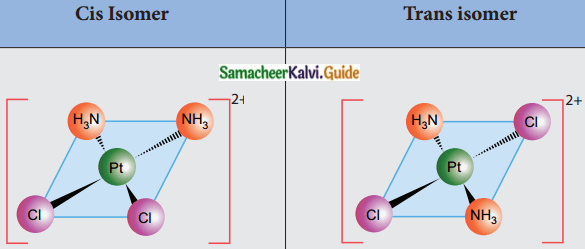Question 2.
Explain geometrical isomerism in octahedral complexes.

• Octahedral complexes of the type [MA2B4], [M(XX)2B2] show Cis- trans isomerism Here A and B are monodentate ligands and XX is a bidentate ligand with two same kind of donor atoms.
• In the above diagram the positions (1,2), (1.3) (1,4) (1,5), (2,3) (2,5), (2,6) (3,4) (3,6), (4,5), (4,6) and (5,6) are identical and if two similar groups are present in any one of these positions, the isomer is called as Cis isomer.
• Similarly, positions (1, 6), (2,4) and (3, 5) are identical and if two similar groups are present in these positions, the isomer is called as trans-isomer.
(ex) [Co (NH3)4Cl2]+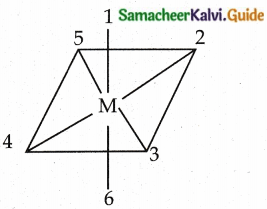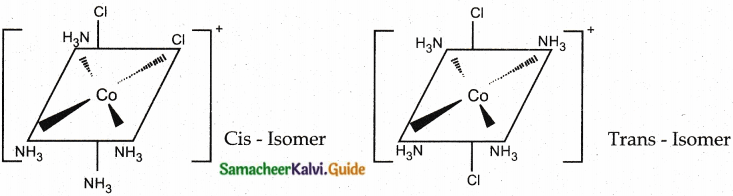Question 3.
Write about facial and meridional isomers.

• Octahedral complex of the type [MA3B3] shows geometrical isomerism
• If the three similar ligands (A) are present in the corners of one triangular face of the octahedron and the other three ligands (B) are present in the opposing triangular face, then the isomer is called as a facial isomer (fac isomer)
• If the three similar ligands are present around the meridian which is an imaginary semicircle from one apex of the octahedron to the opposite apex, the isomer is called as a meridional isomer (mer isomer)
• This is called meridional because each set of ligands can be regarded as lying on a meridian of an octahedron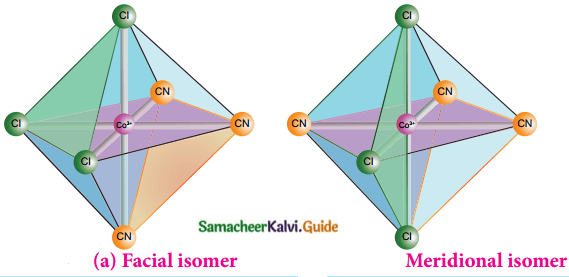Question 4.
In a tetrahedral crystal field, draw the figure to show splitting of d-orbitals (PTA – 6)Question 5.
Write the use of complex formation in photography.
In photography, when the developed film is washed with sodium thiosulphate solution (hypo), the negative film gets fixed. Undecomposed AgBr forms a soluble complex called
sodiumdithiosulphatoargentate(I) which can be easily removed by washing the film with water.
AgBr + 2Na2S2O3 → Na3[Ag (S2O3)2] + 2NaBr

Question 6.
Write the use of metal complexes in biological systems.

• RBC is composed of heme group which is a complex of Fe2+ and Porphyrin ligand. It carries oxygen from lungs to tissues.
• Chlorophyll, a green pigment present in green plants, algae is a complex of Mg2+ and corrin ring, a modified porphyrin ligand. It is responsible for the photosynthesis and the conversion of CO2 and water into carbohydrates and oxygen.
• The only vitamin with a metal ion Vitamin B12 (Cyanocobalamine) is a complex of Co+ and porphyrin ligand.
• Many enzymes which regulate biological processes are metal complexes.
(ex) Carboxypeptidase is a protease enzyme that hydrolytic enzyme important in digestion is a zinc complex with protein-ligand.

Question 7.
How is Cisplatin used as an antitumour drug in cancer treatment?

• C is platin is a square planar coordination complex Cis – [Pt (NH3)2 Cl2]
• It is a platinum-based anti-cancer drug
• This drug undergoes hydrolysis and reacts with DNA to produce various cross-links.
• These cross-links hinder the DNA replication and transcription, which results in cell growth inhibition and ultimately cell death.
• It also cross-links with cellular proteins and inhibits mitosis.

IX. Five Mark Questions

Question 1.
How are coordination complexes classified?
Coordination complexes are classified into two types.
I. Classification based on the net charge on the complex
A coordination compound in which the complexion
(i) Carries a net positive charge is called a cationic complex. (Ex). [Ag(NH3)2]+
(ii) Carries a net negative charge is called an anionic complex. (Ex). [Ag(CN)2]
(iii) Bears with no net charge is called a neutral complex. (Ex). [Ni(CO)4]

II. Classification based on kind of ligands.
A coordination compound in which
(i) The central metal ion/ atom is coordinated to only one kind of ligand is called a homoleptic complex. (Ex). [Ag(NH3)2]+
(ii) The central metal ion/ atom is coordinated to more than one kind of ligands is called a heteroleptic complex. (Ex). [Co (NH3)5 Cl]2+

Question 2.
Write the steps involved in the IUPAC nomenclature of coordination compounds.
i) The cation is named first, followed by the anion regardless of whether the ion is simple or complex.
ii) The simple ions are named as in other ionic compounds.
iii) To name a complexion, the ligands are named first followed by the central metal atom/ ion. If more than one ligand is present they are named in alphabetical order.

(a) Naming the ligands:

• Name of anionic ligands end with the letter ‘O’.
Name of cationic ligands end with ‘ium’.
• Neutral ligands are usually called with their molecular names with fewer exception namely H2O (aqua), NH3 (ammine) etc.,
• K-term is used to denote an ambidentate ligand in which more than one coordination mode is possible.
• If the coordination entity contains more than one ligand of a particular type, the multiples of ligand (2,3,4, …..) is indicated by adding Greek prefixes (di,tri,tetra ….) to the name of the ligand.
• If the name of the ligand contains a Greek prefix (eg. ethylene diamine) alternate prefixes (bis, tris, tetrakis ) can be used.
• These numerical prefixes are not taken into account for alphabetising the name of ligands.

(b) Naming the central metal atom/ion.

• In cationic/neutral complexes, the element name is used as such for naming the central metal atom/ion.
• In anionic complexes a suffix ‘ate’ is used along with the element name or its Greek or Latin name if any.Question 3.
Explain structural isomerism exhibited by coordination compounds.

• The coordination compounds with same formula, but have different connections among their constituent atoms are called structural isomers or constitutional isomers.
• It is of four types.

This type arises when an ambidentate ligand is bonded to the central metal atom/ion through either of its two different donor atoms.
(ex). [Co (NH3)5 NO2]2+ – nitritoK-N ligand N – attached.
[Co (NH3)5 ONO]2+ – nitrito K-O ligand O – attached.

ii) Coordination isomers:
This type arises in the coordination compounds having both the cation and anion as complex ion.

The interchange of one or more ligands between the cationic and the anionic coordination entities result in different isomers.
(ex). [Co (NH3)6] [Cr (CN)6] &
[Cr (NH3)6] [CO (CN)6]

iii) Ionisation isomers:

• This type arises when an ionisable counter ion itself can act as a ligand.
• The exchange of such counterions with one or more ligands in the coordination entity will result in ionisation isomers.
• These isomers will give different ions in solution.
(ex). [Co (NH3)4 Br2] Cl &
[Co (NH3)4 Cl Br] Br.

iv) Solvate isomers:
This arises by the exchange of free solvent molecules such as water, ammonia, alcohol etc., in the crystal lattice with a ligand in the coordination entity.

If the solvent molecule is water, these isomers are called hydrate isomers. (ex).[Cr(H20)6]Cl3;
[Cr (H2O)5 Cl] Cl2 H2O;
[Cr (H2O)4 Cl2] Cl 2H2O.

Question 4.
Explain the main assumption of VBT of coordination compounds (PTA – 1)

• The ligand → Metal bond in coordination complex is an aicnl in nature, it is formed by sharing ol electrons between theienlrul metal atom and the ligand.
• Each ligand should have at least one filled orbital containing a lone pair of electrons.
• To accept the electron pairs donated by the ligands, the central metal ion must contain vacant orbitals equal to its coordination number.
• This vacant orbital of central metal atom undergo hybridisation.
• The vacant hybridized orbitals of central metal ion linearly overlap with filled orbital of the ligands forming coordinate covalent sigma bonds.
• The hybridized orbitals are directional and give definite geometry to the complexion.
• In octahedral complexes, if (n-1) d orbitals are involved in hybridisation, they are called inner orbital complexes or low spin complexes or spin paired complexes. If nd orbitals are involved in hybridisation, they are called as outer orbital complexes, or high spin complexes or spin-free complexes.
• If the metal ion contains unpaired electrons the complex is paramagnetic, if the electrons are paired, the complex is diamagnetic.
• Ligands such as CO, CN, en and NH3 which cause pairing of electrons are called strong field ligands.
• Ligands such as, F, Cl, Br which do not cause pairing of electrons are called weak field ligands.
• Greater the overlapping between the ligand orbitals and the hybridised metal orbital, greater is the bond strength.
• The relation between crystal field splitting energies of octahedral and tetrahedral field is $$\Delta t=\frac{4}{9} \Delta 0$$

Question 5.
Write the salient features of Crystal Field Theory.

• Crystal field theory assumes that the bond between the ligand and the central metal atom is purely ionic, ie. the bond is formed due to the electrostatic attraction between the electron-rich ligand and the electron-deficient metal.
• In case of charged metal ions or ligands, they are considered as point charges and in case of neutral metal atoms or ligands they are considered as electric dipoles.
• The complex formation is considered as the following series of hypothetical steps.

Step-1:
In an isolated gaseous state, all the five ‘d’ orbitals of the central metal ion are degenerate. Initially, the ligands form a spherical field of negative charge around the metal. In this field the energies of all the five d orbitals will increase due to the repulsion between the electrons of the metal and the ligand.

Step-2:
The ligands are approaching the metal atom in actual bond directions. In an octahedral field the central metal ion is located at the origin and the six ligands are coming from +x, -x, +y, -y, +z and -z directions.
Orbitals lying along the axes dx²-y² and d (eg) will experience strong repulsion and raise in energy to a greater extent than the orbitals with lobes directed between the axes (dxy/ dyz, dzx) (t2g). This splitting of degenerated orbitals into two sets is called crystal field splitting.

Step-3:
Upto this point the complex formation would not be favoured. When the ligands approach further, there will be an attraction between the negatively charged electron and the positively charged metal ion resulting in a net decrease in energy. This decrease in energy is the driving force for the complex formation.

In the case of the tetrahedral complex, none of these orbital is directly pointing to the ligands. The dxy, dyz, dzx (t2g) orbitals are nearer to the ligands and hence they interact to a greater extent with the ligand orbitals, therefore dxy, dyz, dzx (t2g) orbitals become high energy orbitals than dx²-y² and d (eg) orbitals in a tetrahedral complex.
Thus the mode of splitting of d orbitals in an octahedral complex is just the reverse of that observed in a tetrahedral complex.Question 6.
Explain the classification of metallic carbonyls.
Metallic carbonyls are classified in two different ways.

I. Classification based on the number of metal atoms present
a) Mononuclear carbonyls:
These contain only one metal atom.
(ex). [Ni (CO)4]

b) Polynuclear carbonyls
These contain two or more metal atoms. They may be homonuclear or heteronuclear.
(ex). [Co2 (CO)8]; [MnCo (CO)9]

II. Classification based on the structure:
a) Non bridged metal carbonyls.
These do not contain any bridging carbonyl ligands.
Those contain only terminal carbonyls.
(ex)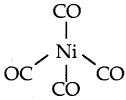Those contain terminal carbonyls and metal-metal bonds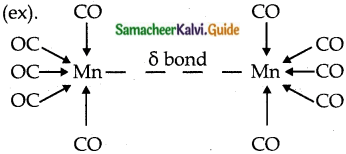b) Bridged carbonyls:
These contain one or more bridging carbonyls along with terminal carbonyl ligands and one or more metal-metal bonds, (ex).Question 7.
Write the IUPAC names for the following complexes. (MARCH 2020)

1. [Co (NH3)5Cl]2+
2. K3 [Fe (C2O4)3]
3. [Co <NH3)6] [Cr(CN)6]
4. [Cr (H2O)4 Cl2] Cl 2H2O
5. [Ft (Py)4] [Pt Cl4]
6. [Zn(NCS)4]2-
7. [Ag(NH3)2]2+

1. Pentaamminechloridocobalt (III)ion
2. Potassium trioxalato ferrate (III)
3. Hexaammine Cobalt (III) hexa cyanido K-C chromate (III)
4. Tetra aqua dichlorido chromium (III) chloride dihydrate
5. Tetrapyridine platinum (II) tetra chloridoplatinate (II)
6. Tetrathiocyanato – kN Zincate (II) ion
7. Diammine Silver (I) ionQuestion 8.
Write the formula for the following coordination compounds.

1. Tris (ethylenediamine) Chromium (III) Chloride
2. Potassium tetracyanido K-C nickelate (II)
3. Ammine bromido chlorido nitrito – kN platinate (II) ion
4. Dichlorido bis (ethane -1, 2 – diamine) Platinum (IV) nitrate
5. Hexa aqua manganese (II) Phosphate

1. [Cr (en)3] Cl3
2. K2[Ni(CN)4]
3. [Pt (NH3) BrCl No2]
4. [Pt (en)2 Cl2] (N03)2
5. [Mn (H2O)6]3 (PO4)2

Question 9.
What will be the correct order for the wavelengths of absorption in the visible region and explain for the following (PTA – 3)
[Ni(NO2)6]4-; [Ni(NH3)6]2+; [Ni(H2O)6]2+
The central metal ion in all three complexes is the same. Therefore, absorption in the visible region depends on the ligands. The order in which the CFSE values of the ligands increases in the spectrochemical series is as follows:
H2O < NH3 < NO2
Thus, the amount of crystal-field splitting observed will be in the following order.
0(H2O) < ∆0(NH3) < ∆0(NO2)
Hence, the wavelengths of absorption in the visible region will be in the order.
[Ni(H2O)6]2+ >[Ni(NH3)6]2+ > [Ni(NO2)6]4-

Question 10.
Answer all the questions for the complex [Fe(en)2Cl2]Cl2 (PTA -6)

1. Oxidation number of Fe
2. Hybridisation and shape
3. Magnetic behaviour
4. Number of geometric isomers
5. Whether there may be optical isomer also?
6. IUPAC name